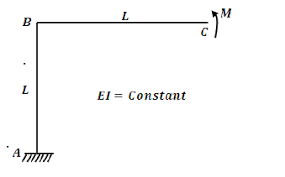## How to Calculate and Solve for Horizontal Deflection |Irrigation Water RequirementThe image above represents horizontal deflection.

To compute for horizontal deflection, seven essential parameters are needed and these parameters are Deflection Lag Factor (Dt), Bedding Angle Factor (K), Soil Load per Unit Length (W), Modulus of Elasticity of Tubing (E), Moment of Inertia of Tubing (I), Mean Radius of Tubing (r) and Modulus of Soil Reaction (E’).

The formula for calculating horizontal deflection:

Δx = DtKW / [EI/r³] + 0.061E’

Where:

Δx = Horizontal Deflection
Dt = Deflection Lag Factor
K = Bedding Angle Factor
W = Soil Load per Unit Length
E = Modulus of Elasticity of Tubing
I = Moment of Inertia of Tubing
r = Mean Radius of Tubing
E’ = Modulus of Soil Reaction

Let’s solve an example;
Find the horizontal deflection when the deflection lag factor is 6, the bedding angle factor is 4, the soil load per unit length is 9, the modulus of elasticity of tubing is 10, the moment of inertia of tubing is 2, the mean radius of tubing is 11 and the modulus of soil reaction is 8.

This implies that;

Dt = Deflection Lag Factor = 6
K = Bedding Angle Factor = 4
W = Soil Load per Unit Length = 9
E = Modulus of Elasticity of Tubing = 10
I = Moment of Inertia of Tubing = 2
r = Mean Radius of Tubing = 11
E’ = Modulus of Soil Reaction = 8

Δx = DtKW / [EI/r³] + 0.061E’
Δx = (6)(4)(9) / [(10)(2)/(11)³] + 0.061(8)
Δx = 216 / [20/1331] + 0.488
Δx = 216 / [0.015] + 0.488
Δx = 216 / 0.50
Δx = 429.40

Therefore, the horizontal deflection is 429.40.